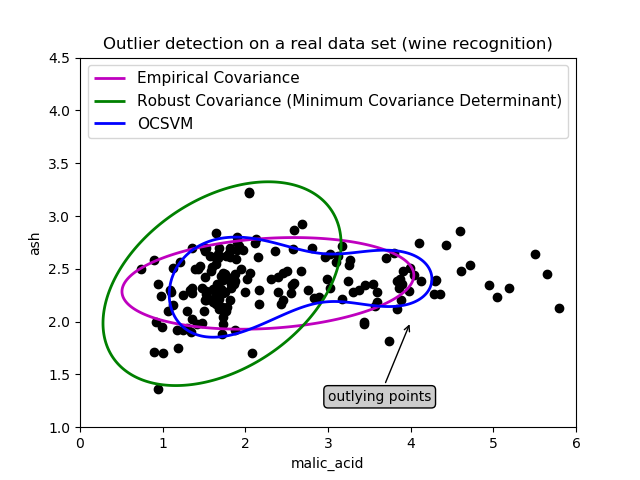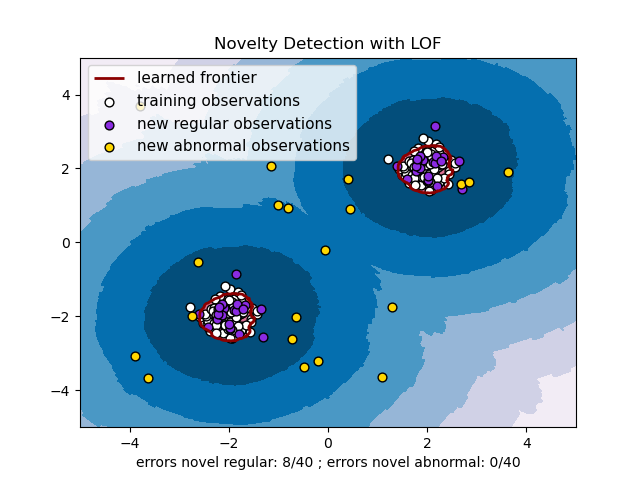# sklearn.svm.OneClassSVM¶

class sklearn.svm.OneClassSVM(*, kernel='rbf', degree=3, gamma='scale', coef0=0.0, tol=0.001, nu=0.5, shrinking=True, cache_size=200, verbose=False, max_iter=-1)

kernel {‘linear’, ‘poly’, ‘rbf’, ‘sigmoid’, ‘precomputed’}, 默认=’rbf’

degree 整数型，默认=3

gamma 浮点数或者{‘scale’, ‘auto’} , 默认=’scale’

coef0 浮点数，默认=0.0

tol 浮点数，默认=1e-3

nu 浮点数，默认=0.5

shrinking 布尔值，默认=True

cache_size 浮点数，默认=200

verbose 布尔值，默认=False

max_iter 整数型，默认=-1

support_ 形如(n_SV,)的数组

support_vectors_ 形如(n_SV, n_features)的数组

dual_coef_ 形如(n_class-1, n_SV)的数组

coef_ 形如(n_class * (n_class-1) / 2, n_features)的数组

intercept_ 形如(n_class * (n_class-1) / 2,)的数组

offset_ 浮点型

fit_status_ 整数型

>>> from sklearn.svm import OneClassSVM>>> X = [, [0.44], [0.45], [0.46], ]>>> clf = OneClassSVM(gamma='auto').fit(X)>>> clf.predict(X)array([-1,  1,  1,  1, -1])>>> clf.score_samples(X)array([1.7798..., 2.0547..., 2.0556..., 2.0561..., 1.7332...])

decision_function(X) 返回各样本点到超平面的函数距离
fit(X, y[, sample_weight]) 根据给定的训练数据拟合支持向量机模型。
fit_predict(X[, y]) 对X拟合并返回X的标签。
get_params([deep]) 获取这个估计器的参数。
predict(X) 在X中对样本进行分类
score_samples(X) 样本的原始评分功能。
set_params(**params) 设置这个估计器的参数。
__init__(*, kernel='rbf', degree=3, gamma='scale', coef0=0.0, tol=0.001, nu=0.5, shrinking=True, cache_size=200, verbose=False, max_iter=-1)

decision_function

X 形如(n_samples, n_features)的数组

dec 形如(n_samples,)的数组

fit(X, y=None, sample_weight=None, **params)

X 形如(n_samples, n_features) 的数组或者稀疏矩阵

sample_weight 形如(n_samples,)的数组，默认=None

y 可忽略

self object

fit_predict(X, y=None)

X 形如(n_samples, n_features) 的数组或者稀疏矩阵
y 可忽略

get_params(deep=True)

deep bool, default = True

params mapping of string to any

predict(X)

X 形如(n_samples, n_features) 或者 (n_samples_test, n_samples_train)的数组或者稀疏矩阵

y_pred 形如(n_sample, )的数组
X中样本的类别标签。
score_samples(X)

X 形如(n_samples, n_features)的数组

score_samples 形如(n_samples, )的数组

set_params(self, **params) 

**params dict

self object

## sklearn.svm.OneClassSVM使用示例¶